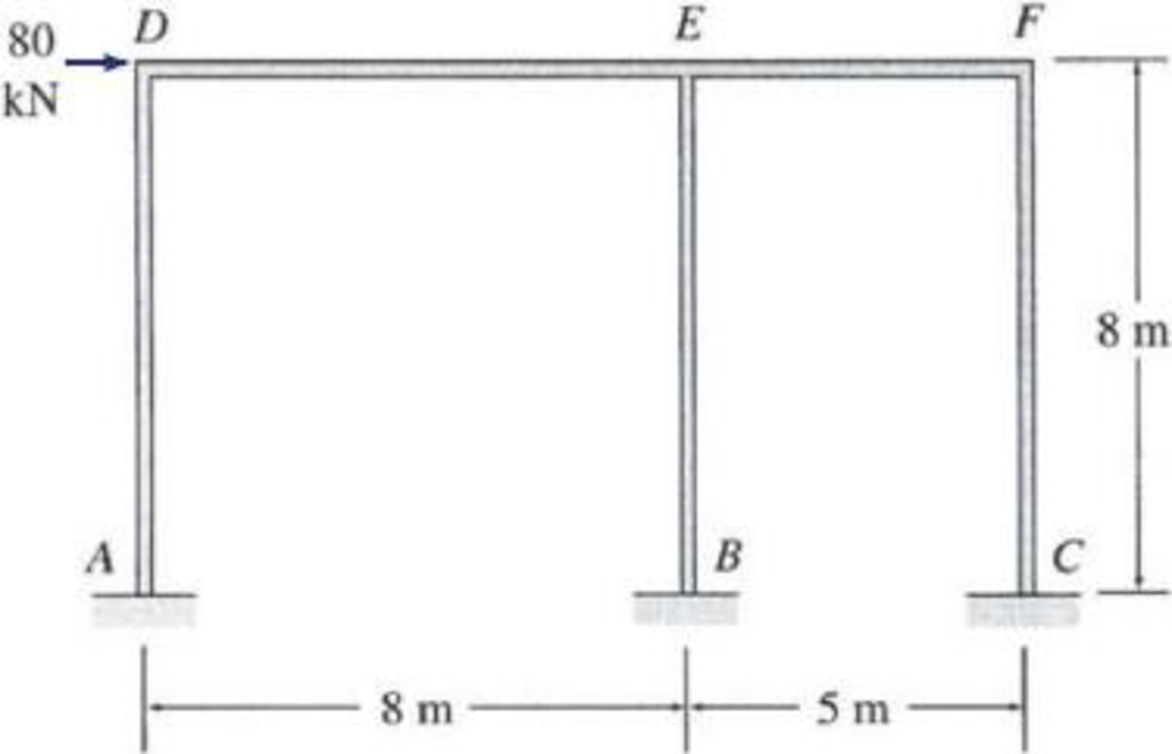# Determine the approximate axial forces, shears, and moments for all the members of the frames show in Figs. P12.6 through P12.13 by using the cantilever method. FIG. P12.8, P12.16

#### Solutions

Chapter
Section
Chapter 12, Problem 16P
Textbook Problem
1 views

## Determine the approximate axial forces, shears, and moments for all the members of the frames show in Figs. P12.6 through P12.13 by using the cantilever method.FIG. P12.8, P12.16To determine

Find the approximate axial forces, shears, and moments for the all members of the frames using cantilever method.

### Explanation of Solution

Given information:

The axial force acting at point D (HD) is 80 kN.

The vertical distance of the member AD, BE, and CF (L) is 8 m.

The horizontal distance of the members AB and DE (l1) is 8 m.

The horizontal distance of the members BC and EF (l2) is 5 m.

Take the counterclockwise moment is positive and clockwise moment is negative.

The axial force in horizontal direction, towards right is positive and towards left side is negative.

The axial force in vertical direction, towards upward is positive and towards downward is negative.

Calculation:

Insert the internal hinges at the midpoints of all the members of the given frame to obtain the simplified frame for approximate analysis.

Draw the simplified frame as in Figure (1).

For the calculation of column axial forces of story of the frame, pass an imaginary section aa through the internal hinges at the midheights of columns AD, BE, and CF.

Draw the free body diagram of the frame portion with the passed imaginary lines as in Figure (2).

Column axial forces:

Above section aa:

Draw the free body diagram of the frame portion above the section aa as in Figure (3).

Determine the location of the centroid using the relation.

x¯=AxA=Ax1+Ax2+Ax33A (1)

Substitute 0 m for x1, 8 m for x2, and 13 m for x3 in Equation (1).

x¯=A(0)+A(8)+A(13)3A=7m

The given lateral load is acting on the frame to the right, therefore the axial force in column AD located to the left of the centroid, must be tensile, whereas the axial force in column CF placed to the right of the centroid, must be compressive.

Consider the axial forces in the columns are to be linearly proportional to their distances from centroid.

Determine the relationship in column axial force between the member AD and BE using the relation.

Substitute 8 m for l1 and 7 m for x¯.

Determine the relationship in column axial force between the member AD and CF using the relation.

Substitute 8 m for l1, 5 m for l2, and 7 m for x¯.

Determine the axial force in the column members AD using equilibrium conditions.

MG=0Q×(l1+l2)HD×L2QBE×l2=0

Substitute 8 m for l1, 5 m for l2, 80 kN for HD, 8 m for L, and 17Q for QBE.

Q×(8+5)80×8217Q×5=013Q5Q7=32086Q=2,240Q=2,24086

Q=26.05kN

The axial force at the column member is QAD=26.05kN().

Determine the axial force in the column members BE.

QBE=26.057=3.72kN()

Determine the axial force in the column members CF.

QCF=6×26.057=22.33kN()

Draw the free body diagram of the frame portion above the section aa with the axial forces in the column members as in Figure (4).

Girder shear and moments:

Consider girder DE.

Determine the shear at upper left end joint D using equilibrium equation.

SD26.05=0SD=26.05kN()

The shear at the girder DE is SDE=26.05kN().

Determine the shear at upper right end joint E using equilibrium equation.

FY=0SDE+SED=0

Substitute 26.05 kN for SDE.

26.05+SED=0SED=26.05kN()

Determine the moment at left end of the girder DE using equilibrium equations.

MDE=SDE×l12

Substitute 26.05 kN for SDE and 8 m for l1.

MDE=26.05×82=104.2kNm=104.2kNm(Clockwise)

Determine the moment at right end of the girder DE using equilibrium equations.

MD=0MDE+SED×l1MED=0

Substitute 104.2kNm for MDE, 26.05 kN for SED, and 8 m for l1.

104.2+26.05×8MED=0MED=104.2kNmMED=104.2kNm(Clockwise)

Consider girder EF.

Determine the shear at left end joint E using equilibrium equation.

FY=0SEF+SEDQBE=0

Substitute 26.05 kN for SED and 3.72kN for QBE.

SEF+26.053.72=0SEF=22.33kNSEF=22.33kN()

Determine the shear at right end joint F using equilibrium equation.

FY=0SEF+SFE=0

Substitute 22.33 kN for SEF.

22.33+SFE=0SFE=22.33kN()

Determine the moment at left end of the girder EF using equilibrium equations.

MEF=SEF×l22

Substitute 22.33 kN for SEF and 5 m for l2.

MEF=22.33×52=55.83kNm=55.83kNm(Clockwise)

Determine the moment at right end of the girder EF using equilibrium equations.

ME=0MEF+SFE×l2MFE=0

Substitute 55

### Still sussing out bartleby?

Check out a sample textbook solution.

See a sample solution

#### The Solution to Your Study Problems

Bartleby provides explanations to thousands of textbook problems written by our experts, many with advanced degrees!

Get Started

Find more solutions based on key concepts
Select an HP-shape for the conditions of Problem 4.6-3. Use Fy=50 ksi. a. Use LRFD. b. Use ASD.

Steel Design (Activate Learning with these NEW titles from Engineering!)

You are to write down your waist size on a piece of paper. If you dont know your waist size, ask your instructo...

Engineering Fundamentals: An Introduction to Engineering (MindTap Course List)

What is Big Data? Give a brief definition.

Database Systems: Design, Implementation, & Management

Prepare a table comparing the Internet access plans in your area. Based on your current Internet usage, which p...

Enhanced Discovering Computers 2017 (Shelly Cashman Series) (MindTap Course List)

What are three typical reasons why companies develop their own information systems?

Systems Analysis and Design (Shelly Cashman Series) (MindTap Course List)

What is an InfoSec program?

Management Of Information Security

If your motherboard supports ECC DDR3 memory, can you substitute non-ECC DDR3 memory?

A+ Guide to Hardware (Standalone Book) (MindTap Course List)